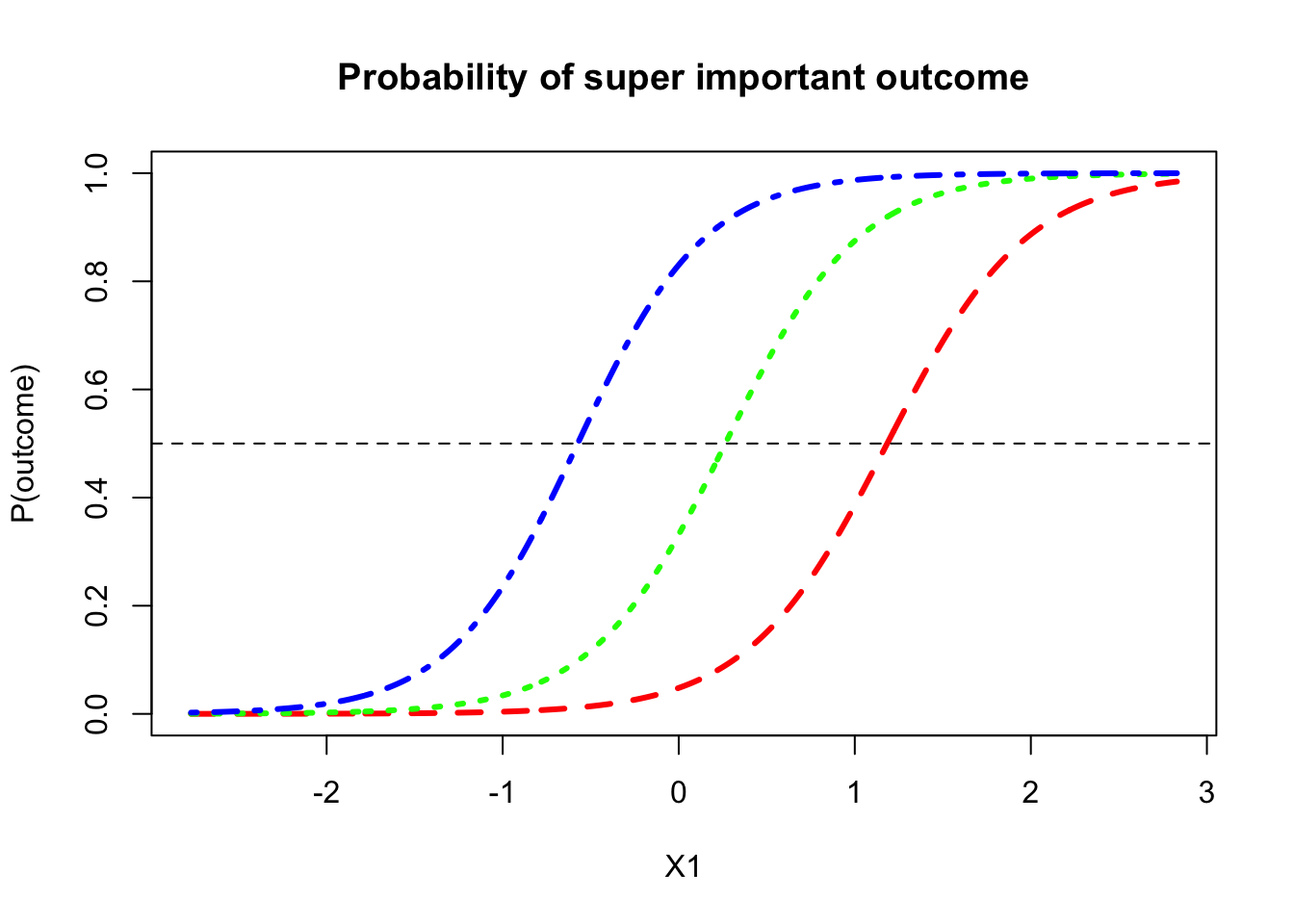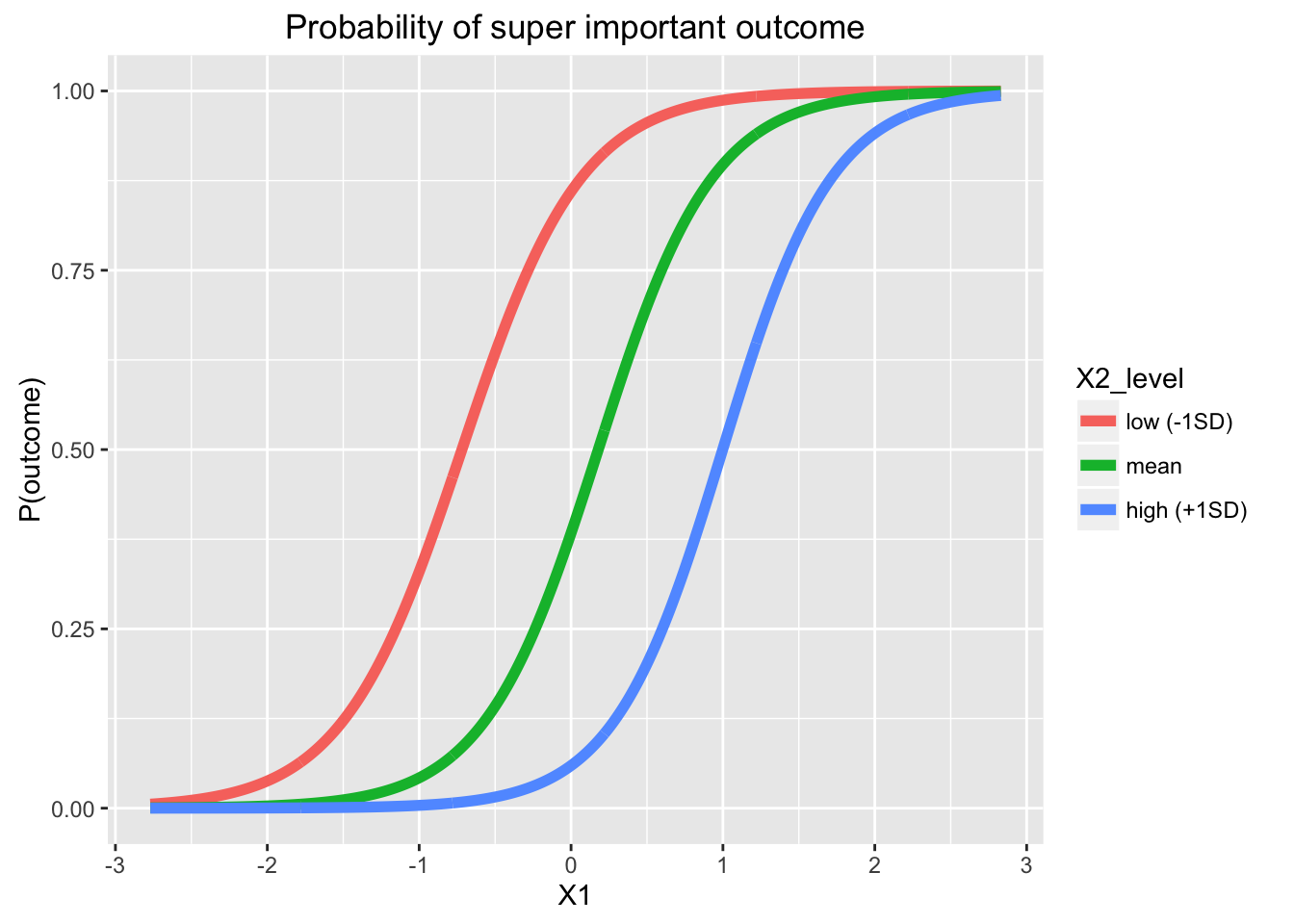# Plotting logistic regression models, part 2

If you haven’t already, check out plotting logistic regression part 1 (continuous by categorical interactions).

All of this code is available on Rose’s github: https://github.com/rosemm/rexamples/blob/master/logistic_regression_plotting_part2.Rmd

# Plotting the results of your logistic regression Part 2: Continuous by continuous interaction

Last time, we ran a nice, complicated logistic regression and made a plot of the a continuous by categorical interaction. This time, we’ll use the same model, but plot the interaction between the two continuous predictors instead, which is a little weirder (hence part 2).

Use the model from the Part 1 code.

Here’s that model:

summary(model)
##
## Call:
## glm(formula = DV ~ (X1 + X2 + group)^2, family = "binomial",
##     data = data, na.action = "na.exclude")
##
## Deviance Residuals:
##      Min        1Q    Median        3Q       Max
## -2.44094  -0.45991   0.04136   0.52301   2.74705
##
## Coefficients:
##             Estimate Std. Error z value Pr(>|z|)
## (Intercept)  -0.5873     0.3438  -1.708 0.087631 .
## X1            2.6508     0.5592   4.740 2.13e-06 ***
## X2           -2.2599     0.4977  -4.540 5.61e-06 ***
## groupb        2.2111     0.5949   3.717 0.000202 ***
## groupc        0.6650     0.4131   1.610 0.107456
## X1:X2         0.1201     0.2660   0.452 0.651534
## X1:groupb     2.7323     1.2977   2.105 0.035253 *
## X1:groupc    -0.6816     0.7078  -0.963 0.335531
## X2:groupb     0.8477     0.7320   1.158 0.246882
## X2:groupc     0.4683     0.6558   0.714 0.475165
## ---
## Signif. codes:  0 '***' 0.001 '**' 0.01 '*' 0.05 '.' 0.1 ' ' 1
##
## (Dispersion parameter for binomial family taken to be 1)
##
##     Null deviance: 412.88  on 299  degrees of freedom
## Residual deviance: 205.46  on 290  degrees of freedom
## AIC: 225.46
##
## Number of Fisher Scoring iterations: 7

And we already saved the coefficients individually for use in the equations last time.

## Calculate probabilities for the plot

Again, we’ll put X1 on the x-axis. That’s the only variable we’ll enter as a whole range.

X1_range <- seq(from=min(data$X1), to=max(data$X1), by=.01)

Next, compute the equations for each line in logit terms.

### Pick some representative values for the other continuous variable

Just like last time, we’ll need to plug in values for all but one variable (X1, which is going on the x-axis of the plot), but this time we’ll pick some representative values for the other continuous predictor, X2, and plug those in to get a separate line for each representative value of X2. Typical choices are high (1SD above the mean), medium (the mean), and low (1SD below the mean) X2. Another great choice is max, median, and min. You can also do 4 or 5 lines instead of just 3, if you want. It’s your party.

Whatever you decide, I recommend checking to make sure the “representative” values you’re plugging in actually make sense given your data. For example, you may not actually have any cases with X2 value 1SD above the mean, in which case maybe you just want to put in max(X2) for the high case instead. It’s kinda weird to plot your models at values that don’t actually exist in your data (cue Twilight Zone music).

summary(data$X2) ## Min. 1st Qu. Median Mean 3rd Qu. Max. ## -3.52900 -0.70950 -0.02922 -0.04995 0.67260 2.38000 (X2_l <- mean(data$X2) - sd(data$X2) ) ##  -1.062196 (X2_m <- mean(data$X2) )
##  -0.0499475
(X2_h <- mean(data$X2) + sd(data$X2) )
##  0.9623013

Now we can go ahead and plug those values into the rest of the equation to get the expected logits across the range of X1 for each of our “groups” (hypothetical low X2 people, hypothetical average X2 people, hypothetical high X2 people).

### If you ran your model in SPSS and you just have the coefficients…

You’ll need to actually calculate the predicted probabilities yourself. Write out the equation for your model and plug in values for everything except the variable that will go on the x-axis.

Remember, these equations need to include every coefficient for the model you ran, whether or not you actually care about plotting them.

In this case, lots of it will just drop out because I’ll be plugging in 0’s for all of the dummy codes (we’re only looking at group a), but I encourage you to keep the terms in your code, so you don’t forget that all of those predictors are still in your model, you’re just holding them constant while you plot. We’re not interested in plotting the categorical predictor right now, but it’s still there in the model, so we need to just pick a group from it and enter the dummy codes for it. The plot will show us the interaction between X1 and X2 for the reference group (for 3-way interactions, you’ll have to wait for part 3!).

X2_l_logits <- b0 +
X1*X1_range +
X2*X2_l +
groupb*0 +
groupc*0 +
X1.X2*X1_range*X2_l +
X1.groupb*X1_range*0 +
X1.groupc*X1_range*0 +
X2.groupb*X2_l*0 +
X2.groupc*X2_l*0

X2_m_logits <- b0 +
X1*X1_range +
X2*X2_m +
groupb*0 +
groupc*0 +
X1.X2*X1_range*X2_m +
X1.groupb*X1_range*0 +
X1.groupc*X1_range*0 +
X2.groupb*X2_m*0 +
X2.groupc*X2_m*0

X2_h_logits <- b0 +
X1*X1_range +
X2*X2_h +
groupb*0 +
groupc*0 +
X1.X2*X1_range*X2_h +
X1.groupb*X1_range*0 +
X1.groupc*X1_range*0 +
X2.groupb*X2_h*0 +
X2.groupc*X2_h*0

# Compute the probibilities (this is what will actually get plotted):
X2_l_probs <- exp(X2_l_logits)/(1 + exp(X2_l_logits))
X2_m_probs <- exp(X2_m_logits)/(1 + exp(X2_m_logits))
X2_h_probs <- exp(X2_h_logits)/(1 + exp(X2_h_logits))

### If you ran your model in R, then you can just use predict()

Easy peasy! And, most importantly, less typing — which means fewer errors. Thanks to John for reminding me of this handy function! You make a new data frame with the predictor values you want to use (i.e. the whole range for X1, group a, and the representative values we picked for X2), and then when you run predict() on it, for each row in the data frame it will generate the predicted value for your DV from the model you saved. The expand.grid() function is a quick and easy way to make a data frame out of all possible combinations of the variables provided. Perfect for this situation!

#make a new data frame with the X values you want to predict
generated_data <- as.data.frame(expand.grid(X1=X1_range, X2=c(X2_l, X2_m, X2_h), group="a") )
head(generated_data)
##          X1        X2 group
## 1 -2.770265 -1.062196     a
## 2 -2.760265 -1.062196     a
## 3 -2.750265 -1.062196     a
## 4 -2.740265 -1.062196     a
## 5 -2.730265 -1.062196     a
## 6 -2.720265 -1.062196     a
summary(generated_data)
##        X1                 X2           group
##  Min.   :-2.77027   Min.   :-1.06220   a:1683
##  1st Qu.:-1.37027   1st Qu.:-1.06220
##  Median : 0.02974   Median :-0.04995
##  Mean   : 0.02974   Mean   :-0.04995
##  3rd Qu.: 1.42973   3rd Qu.: 0.96230
##  Max.   : 2.82973   Max.   : 0.96230
#use predict to get the probability using type='response' rather than 'link'
generated_data$prob <- predict(model, newdata=generated_data, type = 'response') head(generated_data)  ## X1 X2 group prob ## 1 -2.770265 -1.062196 a 0.005614200 ## 2 -2.760265 -1.062196 a 0.005756836 ## 3 -2.750265 -1.062196 a 0.005903074 ## 4 -2.740265 -1.062196 a 0.006053005 ## 5 -2.730265 -1.062196 a 0.006206719 ## 6 -2.720265 -1.062196 a 0.006364312 # let's make a factor version of X2, so we can do gorgeous plotting stuff with it later :) generated_data$X2_level <- factor(generated_data\$X2, labels=c("low (-1SD)", "mean", "high (+1SD)"), ordered=T)
head(generated_data) 
##          X1        X2 group        prob   X2_level
## 1 -2.770265 -1.062196     a 0.005614200 low (-1SD)
## 2 -2.760265 -1.062196     a 0.005756836 low (-1SD)
## 3 -2.750265 -1.062196     a 0.005903074 low (-1SD)
## 4 -2.740265 -1.062196     a 0.006053005 low (-1SD)
## 5 -2.730265 -1.062196     a 0.006206719 low (-1SD)
## 6 -2.720265 -1.062196     a 0.006364312 low (-1SD)

## Plot time!

In base R…

# We'll start by plotting the low X2 group:
plot(X1_range, X2_l_probs,
ylim=c(0,1),
type="l",
lwd=3,
lty=2,
col="red",
xlab="X1", ylab="P(outcome)", main="Probability of super important outcome")

# Add the line for mean X2
lines(X1_range, X2_m_probs,
type="l",
lwd=3,
lty=3,
col="green")

# Add the line for high X2
lines(X1_range, X2_h_probs,
type="l",
lwd=3,
lty=4,
col="blue")

# add a horizontal line at p=.5
abline(h=.5, lty=2)Or, you can do it in ggplot2!

library(ggplot2); library(tidyr)
# first you have to get the information into a long dataframe, which is what ggplot likes :)

# if you calculated the predicted probabilities by writing out the equations, you can combine that all into a dataframe now, and use gather() to make it long
plot.data <- data.frame(low=X2_l_probs, mean=X2_m_probs, high=X2_h_probs, X1=X1_range)
plot.data <- gather(plot.data, key=X2_level, value=prob, -X1) # this means gather all of the columns except X1

# if you used predict(), then everything is already in a nice dataframe for you
plot.data <- generated_data

# check out your plotting data
head(plot.data)
##          X1        X2 group        prob   X2_level
## 1 -2.770265 -1.062196     a 0.005614200 low (-1SD)
## 2 -2.760265 -1.062196     a 0.005756836 low (-1SD)
## 3 -2.750265 -1.062196     a 0.005903074 low (-1SD)
## 4 -2.740265 -1.062196     a 0.006053005 low (-1SD)
## 5 -2.730265 -1.062196     a 0.006206719 low (-1SD)
## 6 -2.720265 -1.062196     a 0.006364312 low (-1SD)
ggplot(plot.data, aes(x=X1, y=prob, color=X2_level)) +
geom_line(lwd=2) +
labs(x="X1", y="P(outcome)", title="Probability of super important outcome")